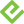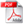# Definitive Guide to DAX, The: Business intelligence for Microsoft Power BI, SQL Server Analysis Services, and Excel, 2nd Edition

## Best Value Purchase

### Book + eBook Bundle

• List Price: \$98.98
• Includes EPUB, MOBI, and PDF
• This eBook includes the following formats, accessible from your Account page after purchase:EPUB The open industry format known for its reflowable content and usability on supported mobile devices.MOBI The eBook format compatible with the Amazon Kindle and Amazon Kindle applications.PDF The popular standard, used most often with the free Adobe® Reader® software.

This eBook requires no passwords or activation to read. We customize your eBook by discreetly watermarking it with your name, making it uniquely yours.

## More Purchase Options

### Book

• List Price: \$54.99
• Usually ships in 24 hours.

### eBook (Watermarked)

• List Price: \$43.99
• Includes EPUB, MOBI, and PDF
• This eBook includes the following formats, accessible from your Account page after purchase:EPUB The open industry format known for its reflowable content and usability on supported mobile devices.MOBI The eBook format compatible with the Amazon Kindle and Amazon Kindle applications.PDF The popular standard, used most often with the free Adobe® Reader® software.

This eBook requires no passwords or activation to read. We customize your eBook by discreetly watermarking it with your name, making it uniquely yours.

### Features

• Only advanced DAX book on the market
• The authors' stellar reputation, which is largely based on high-quality books they have already authored
• The quickly increasing interest in technical content for BI professionals

## Description

• Dimensions: 7-3/8" x 9-1/8"
• Pages: 768
• Edition: 2nd
• Book
• ISBN-10: 1-5093-0697-8
• ISBN-13: 978-1-5093-0697-8

Now expanded and updated with modern best practices, this is the most complete guide to Microsoft’s DAX language for business intelligence, data modeling, and analytics. Expert Microsoft BI consultants Marco Russo and Alberto Ferrari help you master everything from table functions through advanced code and model optimization. You’ll learn exactly what happens under the hood when you run a DAX expression, and use this knowledge to write fast, robust code. This edition focuses on examples you can build and run with the free Power BI Desktop, and helps you make the most of the powerful syntax of variables (VAR) in Power BI, Excel, or Analysis Services. Want to leverage all of DAX’s remarkable capabilities? This no-compromise “deep dive” is exactly what you need.

Perform powerful data analysis with DAX for Power BI, SQL Server, and Excel

·         Master core DAX concepts, including calculated columns, measures, and calculation groups

·         Work efficiently with basic and advanced table functions

·         Understand evaluation contexts and the CALCULATE and CALCULATETABLE functions

·         Perform time-based calculations

·         Use calculation groups and calculation items

·         Use syntax of variables (VAR) to write more readable, maintainable code

·         Express diverse and unusual relationships with DAX, including many-to-many relationships and bidirectional filters

·         Master advanced optimization techniques, and improve performance in aggregations

·         Optimize data models to achieve better compression

·         Measure DAX query performance with DAX Studio and learn how to optimize your DAX

2. If prompted, click Save.
3. Locate the .zip file on your computer. Right-click the file, click Extract All, and then follow the instructions.

## Sample Content

### Sample Pages

Foreword

Introduction to the second edition

Introduction to the first edition

Chapter 1 What is DAX?

Understanding the data model

Understanding the direction of a relationship

DAX for Excel users

Cells versus tables

Excel and DAX: Two functional languages

Iterators in DAX

DAX requires theory

DAX for SQL developers

Relationship handling

DAX is a functional language

DAX as a programming and querying language

Subqueries and conditions in DAX and SQL

DAX for MDX developers

Multidimensional versus Tabular

DAX as a programming and querying language

Hierarchies

Leaf-level calculations

DAX for Power BI users

Chapter 2 Introducing DAX

Understanding DAX calculations

DAX data types

DAX operators

Table constructors

Conditional statements

Understanding calculated columns and measures

Calculated columns

Measures

Introducing variables

Handling errors in DAX expressions

Conversion errors

Arithmetic operations errors

Intercepting errors

Generating errors

Formatting DAX code

Introducing aggregators and iterators

Using common DAX functions

Aggregation functions

Logical functions

Information functions

Mathematical functions

Trigonometric functions

Text functions

Conversion functions

Date and time functions

Relational functions

Conclusions

Chapter 3 Using basic table functions

Introducing table functions

Introducing EVALUATE syntax

Understanding FILTER

Introducing ALL and ALLEXCEPT

Understanding VALUES, DISTINCT, and the blank row

Using tables as scalar values

Introducing ALLSELECTED

Conclusions

Chapter 4 Understanding evaluation contexts

Introducing evaluation contexts

Understanding filter contexts

Understanding the row context

Testing your understanding of evaluation contexts

Using SUM in a calculated column

Using columns in a measure

Using the row context with iterators

Nested row contexts on different tables

Nested row contexts on the same table

Using the EARLIER function

Understanding FILTER, ALL, and context interactions

Working with several tables

Row contexts and relationships

Filter context and relationships

Using DISTINCT and SUMMARIZE in filter contexts

Conclusions

Chapter 5 Understanding CALCULATE and CALCULATETABLE

Introducing CALCULATE and CALCULATETABLE

Creating filter contexts

Introducing CALCULATE

Using CALCULATE to compute percentages

Introducing KEEPFILTERS

Filtering a single column

Filtering with complex conditions

Evaluation order in CALCULATE

Understanding context transition

Row context and filter context recap

Introducing context transition

Context transition in calculated columns

Context transition with measures

Understanding circular dependencies

CALCULATE modifiers

Understanding USERELATIONSHIP

Understanding CROSSFILTER

Understanding KEEPFILTERS

Understanding ALL in CALCULATE

Introducing ALL and ALLSELECTED with no parameters

CALCULATE rules

Chapter 6 Variables

Introducing VAR syntax

Understanding that variables are constant

Understanding the scope of variables

Using table variables

Understanding lazy evaluation

Common patterns using variables

Conclusions

Chapter 7 Working with iterators and with CALCULATE

Using iterators

Understanding iterator cardinality

Leveraging context transition in iterators

Using CONCATENATEX

Iterators returning tables

Solving common scenarios with iterators

Computing averages and moving averages

Using RANKX

Changing calculation granularity

Conclusions

Chapter 8 Time intelligence calculations

Introducing time intelligence

Automatic Date/Time in Power BI

Automatic date columns in Power Pivot for Excel

Date table template in Power Pivot for Excel

Building a date table

Using CALENDAR and CALENDARAUTO

Working with multiple dates

Handling multiple relationships to the Date table

Handling multiple date tables

Understanding basic time intelligence calculations

Using Mark as Date Table

Introducing basic time intelligence functions

Using year-to-date, quarter-to-date, and month-to-date

Computing time periods from prior periods

Mixing time intelligence functions

Computing a difference over previous periods

Computing a moving annual total

Using the right call order for nested time intelligence functions

Using LASTDATE and LASTNONBLANK

Working with opening and closing balances

Understanding periods to date

Understanding FIRSTDATE, LASTDATE, FIRSTNONBLANK, and LASTNONBLANK

Using drillthrough with time intelligence

Working with custom calendars

Working with weeks

Custom year-to-date, quarter-to-date, and month-to-date

Conclusions

Chapter 9 Calculation groups

Introducing calculation groups

Creating calculation groups

Understanding calculation groups

Understanding calculation item application

Understanding calculation group precedence

Including and excluding measures from calculation items

Understanding sideways recursion

Using the best practices

Conclusions

Chapter 10 Working with the filter context

Using HASONEVALUE and SELECTEDVALUE

Introducing ISFILTERED and ISCROSSFILTERED

Understanding differences between VALUES and FILTERS

Understanding the difference between ALLEXCEPT and ALL/VALUES

Using ALL to avoid context transition

Using ISEMPTY

Introducing data lineage and TREATAS

Understanding arbitrarily shaped filters

Conclusions

Chapter 11 Handling hierarchies

Computing percentages over hierarchies

Handling parent/child hierarchies

Conclusions

Chapter 12 Working with tables

Using CALCULATETABLE

Manipulating tables

Using SUMMARIZE

Using CROSSJOIN

Using UNION

Using INTERSECT

Using EXCEPT

Using tables as filters

Implementing OR conditions

Narrowing sales computation to the first year’s customers

Computing new customers

Reusing table expressions with DETAILROWS

Creating calculated tables

Using SELECTCOLUMNS

Creating static tables with ROW

Creating static tables with DATATABLE

Using GENERATESERIES

Conclusions

Chapter 13 Authoring queries

Introducing DAX Studio

Understanding EVALUATE

Introducing the EVALUATE syntax

Using VAR in DEFINE

Using MEASURE in DEFINE

Implementing common DAX query patterns

Using ROW to test measures

Using SUMMARIZE

Using SUMMARIZECOLUMNS

Using TOPN

Using GENERATE and GENERATEALL

Using ISONORAFTER

Using TOPNSKIP

Using GROUPBY

Using NATURALINNERJOIN and NATURALLEFTOUTERJOIN

Using SUBSTITUTEWITHINDEX

Using SAMPLE

Understanding the auto-exists behavior in DAX queries

Conclusions

Introducing expanded tables

Understanding RELATED

Using RELATED in calculated columns

Understanding the difference between table filters and column filters

Using table filters in measures

Understanding active relationships

Difference between table expansion and filtering

Context transition in expanded tables

Understanding ALLSELECTED and shadow filter contexts

ALLSELECTED returns the iterated rows

ALLSELECTED without parameters

The ALL* family of functions

ALL

ALLEXCEPT

ALLNOBLANKROW

ALLSELECTED

ALLCROSSFILTERED

Understanding data lineage

Conclusions

Implementing calculated physical relationships

Computing multiple-column relationships

Implementing relationships based on ranges

Understanding circular dependency in calculated physical relationships

Implementing virtual relationships

Transferring filters in DAX

Transferring a filter using TREATAS

Transferring a filter using INTERSECT

Transferring a filter using FILTER

Implementing dynamic segmentation using virtual relationships

Understanding physical relationships in DAX

Using bidirectional cross-filters

Understanding one-to-many relationships

Understanding one-to-one relationships

Understanding many-to-many relationships

Implementing many-to-many using a bridge table

Implementing many-to-many using a common dimension

Implementing many-to-many using MMR weak relationships

Choosing the right type of relationships

Managing granularities

Managing ambiguity in relationships

Understanding ambiguity in active relationships

Solving ambiguity in non-active relationships

Conclusions

Chapter 16 Advanced calculations in DAX

Computing the working days between two dates

Showing budget and sales together

Computing same-store sales

Numbering sequences of events

Computing previous year sales up to last date of sales

Conclusions

Chapter 17 The DAX engines

Understanding the architecture of the DAX engines

Introducing the formula engine

Introducing the storage engine

Introducing the VertiPaq (in-memory) storage engine

Introducing the DirectQuery storage engine

Understanding data refresh

Understanding the VertiPaq storage engine

Introducing columnar databases

Understanding VertiPaq compression

Understanding segmentation and partitioning

Using Dynamic Management Views

Understanding the use of relationships in VertiPaq

Introducing materialization

Introducing aggregations

Choosing hardware for VertiPaq

Hardware choice as an option

Set hardware priorities

CPU model

Memory speed

Number of cores

Memory size

Disk I/O and paging

Best practices in hardware selection

Conclusions

Chapter 18 Optimizing VertiPaq

Gathering information about the data model

Denormalization

Columns cardinality

Handling date and time

Calculated columns

Optimizing complex filters with Boolean calculated columns

Processing of calculated columns

Choosing the right columns to store

Optimizing column storage

Using column split optimization

Optimizing high-cardinality columns

Disabling attribute hierarchies

Optimizing drill-through attributes

Managing VertiPaq Aggregations

Conclusions

Chapter 19 Analyzing DAX query plans

Capturing DAX queries

Introducing DAX query plans

Collecting query plans

Introducing logical query plans

Introducing physical query plans

Introducing storage engine queries

Capturing profiling information

Using DAX Studio

Using the SQL Server Profiler

Introducing xmSQL syntax

Understanding scan time

Understanding DISTINCTCOUNT internals

Understanding parallelism and datacache

Understanding the VertiPaq cache

Understanding CallbackDataID

Analyzing composite models

Using aggregations in the data model

Conclusions

Chapter 20 Optimizing DAX

Defining optimization strategies

Identifying a single DAX expression to optimize

Creating a reproduction query

Analyzing server timings and query plan information

Identifying bottlenecks in the storage engine or formula engine

Implementing changes and rerunning the test query

Optimizing bottlenecks in DAX expressions

Optimizing filter conditions

Optimizing context transitions

Optimizing IF conditions

Reducing the impact of CallbackDataID

Optimizing nested iterators

Avoiding table filters for DISTINCTCOUNT

Avoiding multiple evaluations by using variables

Conclusions

9781509306978   TOC   5/23/2019